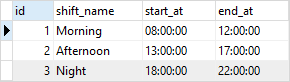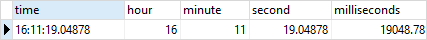# PostgreSQL Tutorial: TIME Data Type

August 2, 2023

Summary: in this tutorial, we will introduce you to the PostgreSQL `TIME` data types and show you some handy functions to handle time values.

## Introduction to PostgreSQL TIME data type

PostgreSQL provides the `TIME` data type that allows you to store the time of day values.

The following statement illustrates how to declare a column with the `TIME` data type:

``````column_name TIME(precision);
``````

A time value may have a precision up to 6 digits. The precision specifies the number of fractional digits placed in the second field.

The `TIME` data type requires 8 bytes and its allowed range is from `00:00:00` to `24:00:00`. The following illustrates the common formats of the `TIME` values:

``````HH:MI
HH:MI:SS
HHMISS
``````

For example:

``````01:02
01:02:03
010203
``````

If you want to use the precision, you can use the following formats:

``````MI:SS.pppppp
HH:MI:SS.pppppp
HHMISS.pppppp
``````

In this form, `p` is the precision. For example:

``````04:59.999999
04:05:06.777777
040506.777777
``````

PostgreSQL actually accepts almost any reasonable `TIME` format including SQL-compatible, ISO 8601, etc.

## PostgreSQL TIME example

We often use the `TIME` data type for the columns that store the time of day only e.g., the time of an event or a shift. Consider the following example.

First, create a new table named `shifts` by using the following `CREATE TABLE` statement:

``````CREATE TABLE shifts (
id serial PRIMARY KEY,
shift_name VARCHAR NOT NULL,
start_at TIME NOT NULL,
end_at TIME NOT NULL
);
``````

Second, insert some rows into the `shifts` table:

``````INSERT INTO shifts(shift_name, start_at, end_at)
VALUES('Morning', '08:00:00', '12:00:00'),
('Afternoon', '13:00:00', '17:00:00'),
('Night', '18:00:00', '22:00:00');
``````

Third, query data from the `shifts` table:

``````SELECT * FROM shifts;
``````## PostgreSQL TIME with time zone type

Besides the `TIME` data type, PostgreSQL provides the `TIME with time zone` data type that allows you to store and manipulate the time of day with time zone.

The following statement illustrates how to declare a column whose data type is `TIME with time zone`:

``````column TIME with time zone
``````

The storage size of the `TIME with time zone` data type is 12 bytes that allow you store a time value with the time zone that ranges from `00:00:00+1459` to `24:00:00-1459`.

The following illustrates the `TIME with time zone` values:

``````04:05:06 PST
04:05:06.789-8
``````

## Handling PostgreSQL TIME values

### Getting the current time

To get the current time with time zone, you use the `CURRENT_TIME` function as follows:

``````SELECT CURRENT_TIME;
``````
``````timetz
--------------------
00:51:02.746572-08
(1 row)
``````

To get the current time with a specific precision, you use the `CURRENT_TIME(precision)` function:

`````` SELECT CURRENT_TIME(5);
``````
``````   current_time
-------------------
00:52:12.19515-08
(1 row)
``````

Notice that without specifying the precision, the `CURRENT_TIME` function returns a time value with the full available precision.

To get the local time, you use the `LOCALTIME` function:

``````SELECT LOCALTIME;
``````
``````      localtime
-----------------
00:52:40.227186
(1 row)
``````

Similarly, to get the local time with a specific precision, you use the `LOCALTIME(precision)` function:

`````` SELECT LOCALTIME(0);
``````
`````` localtime
----------
00:56:08
(1 row)
``````

### Converting time to a different time zone

To convert time to a different time zone, you use the following form:

``````[TIME with time zone] AT TIME ZONE time_zone
``````

For example, to convert the local time to the time at the time zone UTC-7, you use the following statement:

``````SELECT LOCALTIME AT TIME ZONE 'UTC-7';
``````
``````      timezone
--------------------
16:02:38.902271+07
(1 row)
``````

### Extracting hours, minutes, seconds from a time value

To extracting hours, minutes, seconds from a time value, you use the `EXTRACT` function as follows:

``````EXTRACT(field FROM time_value);
``````

The field can be the hour, minute, second, milliseconds as shown in the following example:

``````SELECT
LOCALTIME,
EXTRACT (HOUR FROM LOCALTIME) as hour,
EXTRACT (MINUTE FROM LOCALTIME) as minute,
EXTRACT (SECOND FROM LOCALTIME) as second,
EXTRACT (milliseconds FROM LOCALTIME) as milliseconds;
``````### Arithmetic operations on time values

PostgreSQL allows you to apply arithmetic operators such as +, -, and * on time values and between time and interval values.

The following statement returns an interval between two time values:

``````SELECT time '10:00' - time '02:00' AS result;
``````
``````  result
----------
08:00:00
(1 row)
``````

The following statement adds 2 hours to the local time:

`````` SELECT LOCALTIME + interval '2 hours' AS result;
``````
``````    result
-----------------
03:16:18.020418
(1 row)
``````

In this example, the sum of a time value and an interval value is a time value.

In this tutorial, you have learned about the PostgreSQL `TIME` data type and how to handle time values using time-related functions.